# PSAT Score Release, December Scholarships & more!# Hello, The College Map!

 table div table+table+table+table div table{width:100%;padding:0}table div table+table+table+table div table img{width:96.23%;padding:0;float:none}table div table+table+table+table div table td{width:100%;padding:0 1.88% 18px}/* styles */## PSAT SCORE REPORTING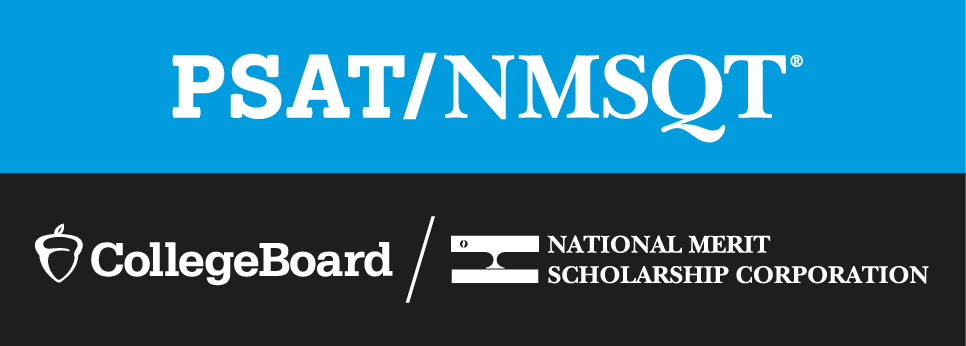/* styles */ PSAT scores for Florida will be released on Tuesday, December 11th. Please take a moment to look up your scores in your collegeboard account and report them to your College Map consultant or update them in your CPP accounts under testing.
 table div table+table+table+table+table+table+table+table div table{width:100%;padding:0}table div table+table+table+table+table+table+table+table div table img{width:96.23%;padding:0;float:none}table div table+table+table+table+table+table+table+table div table td{width:100%;padding:0 1.88% 18px}/* styles */## December ScholarshipsDo you like making a difference in your school or community? If you do, DoSomething has many campaigns to make a difference, and for participating, you will be entered to win a scholarship! Below are campaigns from DoSomething that are accepting entries in December.

 table div table+table+table+table+table+table+table+table+table+table+table div table{width:100%;padding:0}table div table+table+table+table+table+table+table+table+table+table+table div table img{width:96.23%;padding:0;float:none}table div table+table+table+table+table+table+table+table+table+table+table div table td{width:100%;padding:0 1.88% 18px}/* styles */## Are you interested in Driving School?B.R.A.K.E.S. Teen Pro-Active Driving School is a FREE* four-hour program that partners your teen with professional driving instructors to teach the skills of defensive driving in a controlled, hands on environment. This life-saving experience is fun for your teen and they will leave with a great level of confidence. Car crashes are the #1 killer among teens. Together we can change this.

## UPCOMING DATES!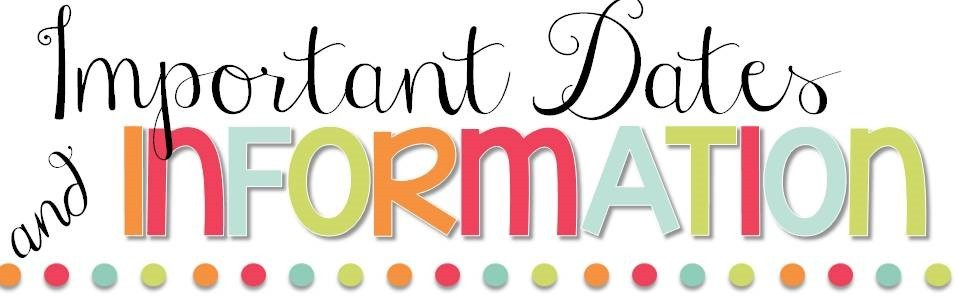table div table+table+table+table+table+table+table+table+table+table+table+table+table+table+table+table div table{width:100%;padding:0}table div table+table+table+table+table+table+table+table+table+table+table+table+table+table+table+table div table img{width:96.23%;padding:0;float:none}table div table+table+table+table+table+table+table+table+table+table+table+table+table+table+table+table div table td{width:100%;padding:0 1.88% 18px}/* styles */SENIORS with late December and January application deadlines, this is extremely important!

All schools will be closed late December through early January though dates may vary. Please pretend all applications are due by December 19th prior to school closings!

In order to meet your deadlines you must have requested transcripts from your counselor at your high school in time for them to be sent in advance of the holiday break. Remember most schools want all materials including transcripts prior to their deadlines.

 table div table+table+table+table+table+table+table+table+table+table+table+table+table+table+table+table+table+table div table{width:100%;padding:0}table div table+table+table+table+table+table+table+table+table+table+table+table+table+table+table+table+table+table div table img{width:96.23%;padding:0;float:none}table div table+table+table+table+table+table+table+table+table+table+table+table+table+table+table+table+table+table div table td{width:100%;padding:0 1.88% 18px}/* styles */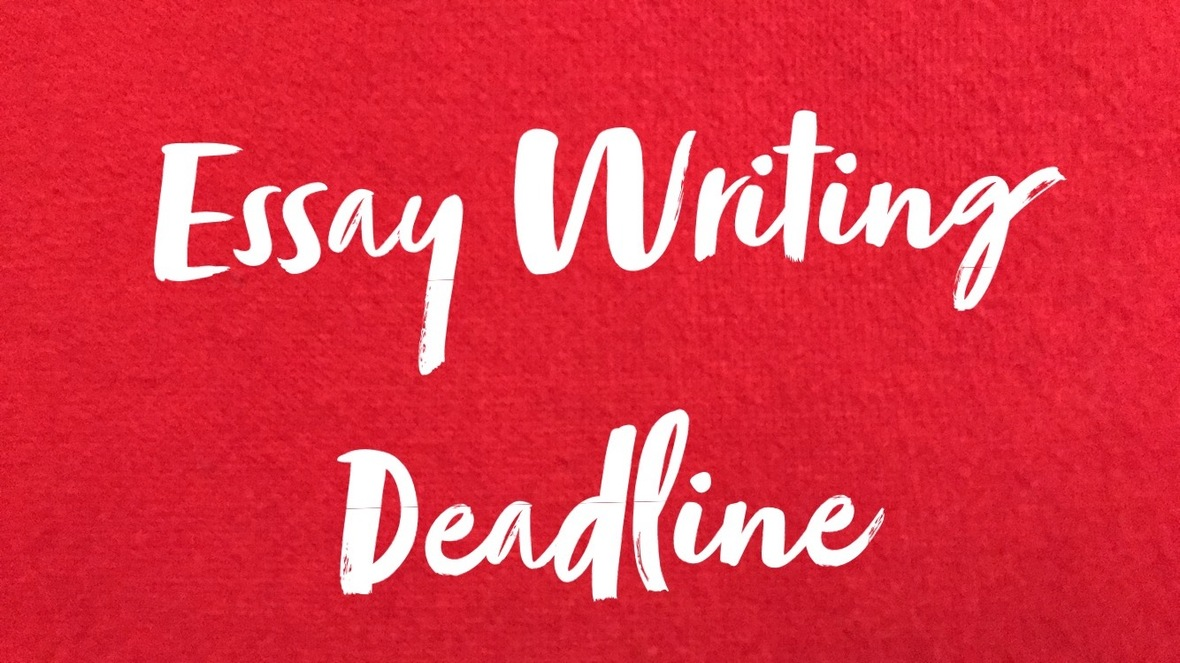DEADLINE for reviews/edits for essay drafts, personal statements, and supplemental essays must be submitted to Julie Mitchell, NO LATER than December 10th! THIS IS A MUST! She has to have time to get the edits back to you in time to submit all your December and January applications prior to the break and preferably by 12/19/19. Prior to the holiday break our counselors are available as always to help ensure our students meet these upcoming deadlines.

 table div table+table+table+table+table+table+table+table+table+table+table+table+table+table+table+table+table+table+table+table div table{width:100%;padding:0}table div table+table+table+table+table+table+table+table+table+table+table+table+table+table+table+table+table+table+table+table div table img{width:96.23%;padding:0;float:none}table div table+table+table+table+table+table+table+table+table+table+table+table+table+table+table+table+table+table+table+table div table td{width:100%;padding:0 1.88% 18px}/* styles */The College Map will be closed beginning Friday, December 21, 2018 and will reopen January 7, 2019.

 table div table+table+table+table+table+table+table+table+table+table+table+table+table+table+table+table+table+table+table+table+table+table div table{width:100%;padding:0}table div table+table+table+table+table+table+table+table+table+table+table+table+table+table+table+table+table+table+table+table+table+table div table img{width:96.23%;padding:0;float:none}table div table+table+table+table+table+table+table+table+table+table+table+table+table+table+table+table+table+table+table+table+table+table div table td{width:100%;padding:0 1.88% 18px}/* styles */## Bright Futures Information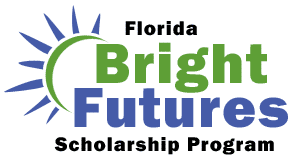/* styles */ Students must submit a completed Florida Financial Aid Application (FFAA) in order to be considered for State Scholarship & Grant Programs. Seniors, please Create a Student Account. After logging into your account, you may proceed to complete the FFAA. The Bright Futures link can answer all of your questions: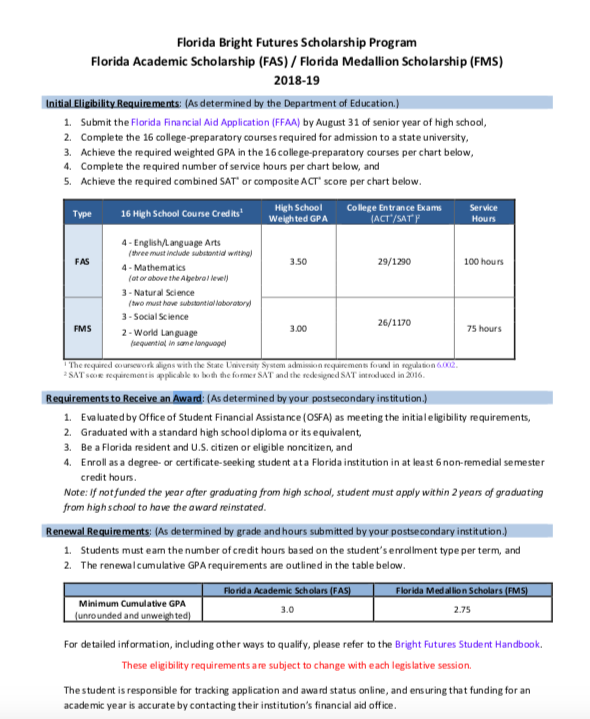table div table+table+table+table+table+table+table+table+table+table+table+table+table+table+table+table+table+table+table+table+table+table+table+table+table+table+table div table{width:100%;padding:0}table div table+table+table+table+table+table+table+table+table+table+table+table+table+table+table+table+table+table+table+table+table+table+table+table+table+table+table div table img{width:96.23%;padding:0;float:none}table div table+table+table+table+table+table+table+table+table+table+table+table+table+table+table+table+table+table+table+table+table+table+table+table+table+table+table div table td{width:100%;padding:0 1.88% 18px}/* styles */## JUNIORS/CLASS OF 2020 SUMMER DATES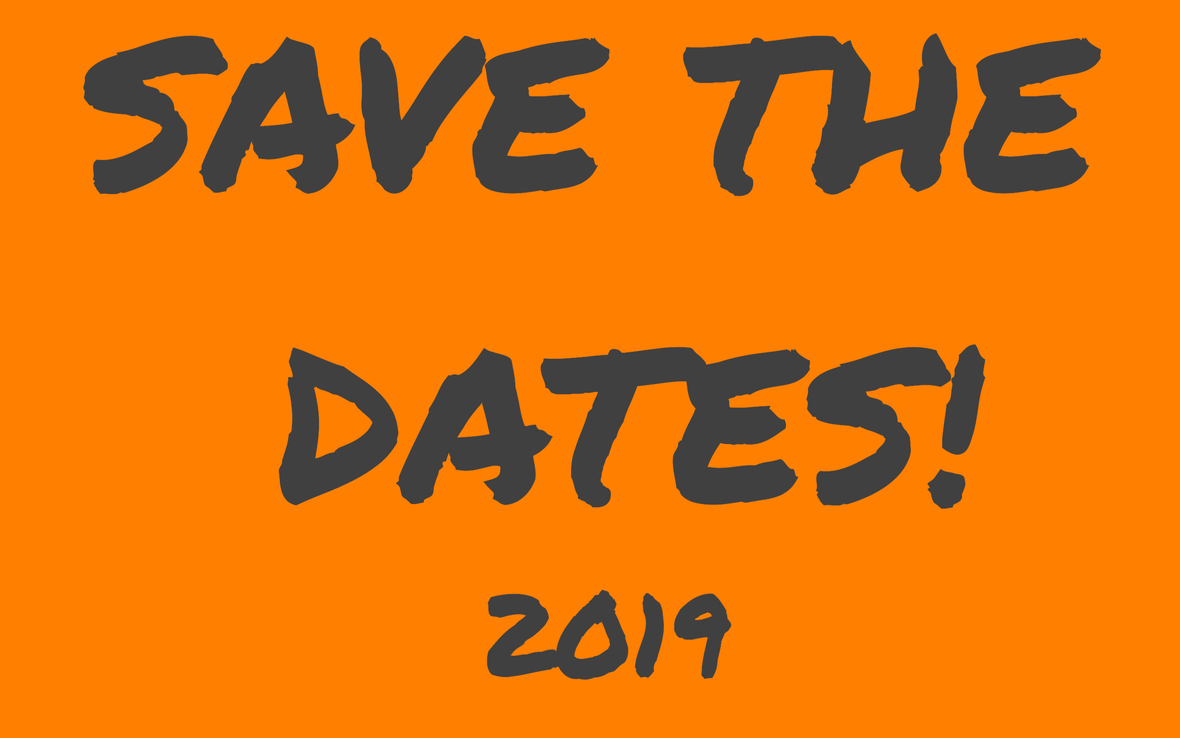/* styles */ ** JUNIORS/CLASS OF 2020 ESSAY WORKSHOP & SENIOR BOOT CAMPS DATES! Writing workshops will be held Saturday, May 18, 2019 and Saturday, June 1, 2019 from 10:00 a.m.until Noon. Students in the class of 2020 must attend one of these two workshops. Please mark your calendars and plan accordingly. Senior Boot Camps for the class of 2020 will be held for two weeks and we also ask that you plan your summer calendar with this in mind and attend all three days of at atleast one of the following weeks: July 23, 24 & 25 or July 30, 31 & August 1st. All dates will be 10:00 a.m. - 6:00 p.m.
 table div table+table+table+table+table+table+table+table+table+table+table+table+table+table+table+table+table+table+table+table+table+table+table+table+table+table+table+table+table+table+table div table{width:100%;padding:0}table div table+table+table+table+table+table+table+table+table+table+table+table+table+table+table+table+table+table+table+table+table+table+table+table+table+table+table+table+table+table+table div table img{width:96.23%;padding:0;float:none}table div table+table+table+table+table+table+table+table+table+table+table+table+table+table+table+table+table+table+table+table+table+table+table+table+table+table+table+table+table+table+table div table td{width:100%;padding:0 1.88% 18px}/* styles */## 2019 Test Prep Calendars

 /* styles */ Please sign up at our Acuity Scheduler website! See below for our new 2019 calendars. Please register for the ACT at act.org OR SAT at collegeboard.org. Please note there are early registration deadline dates. You can register after the deadline date but a late fee will be required.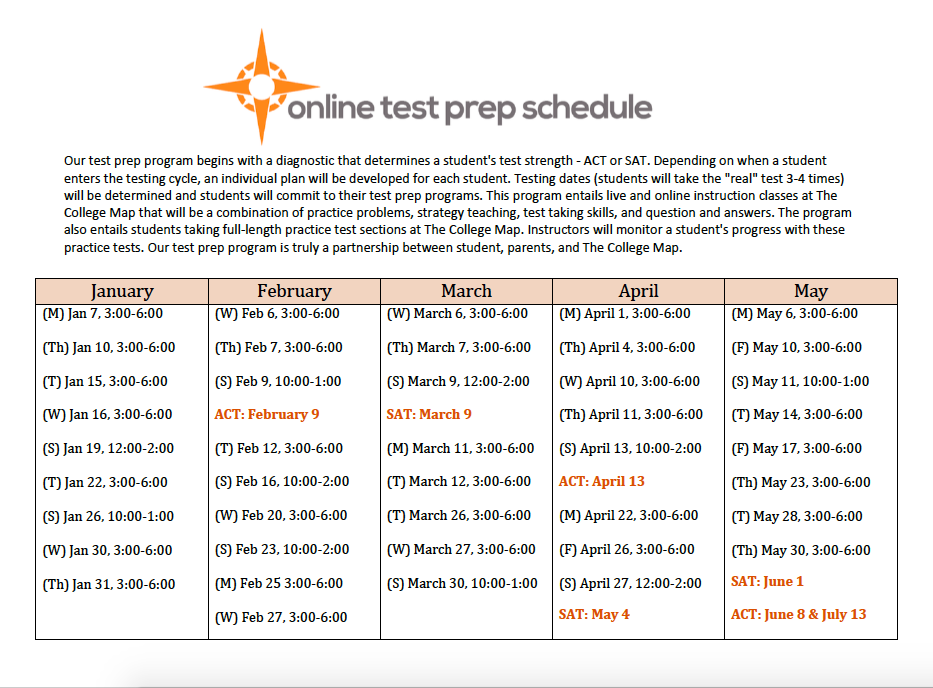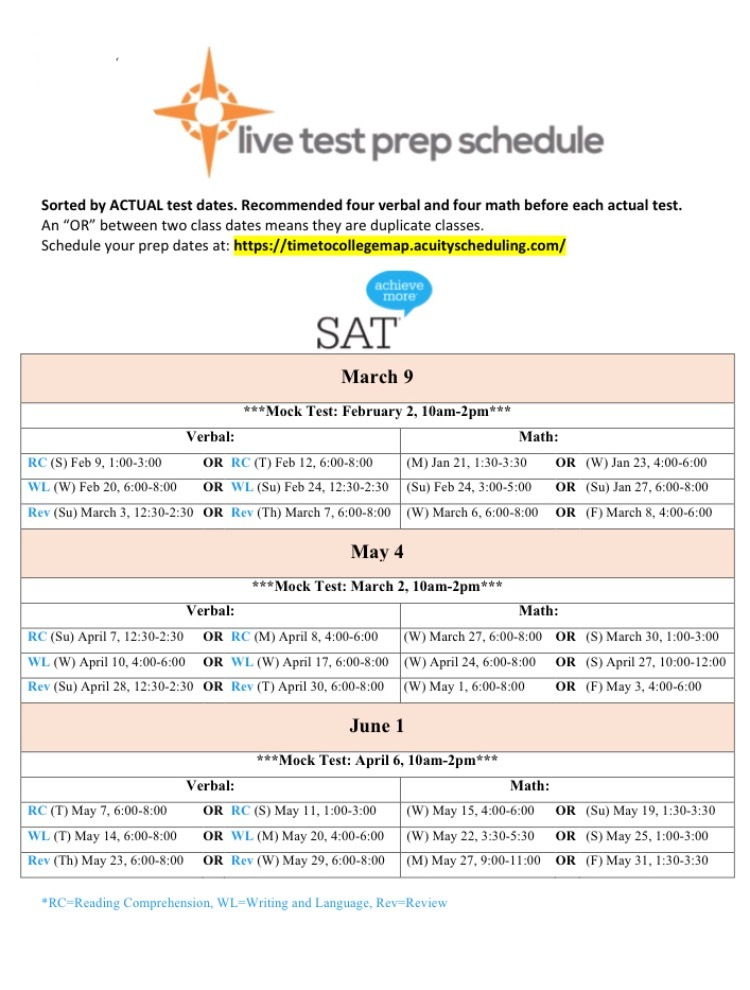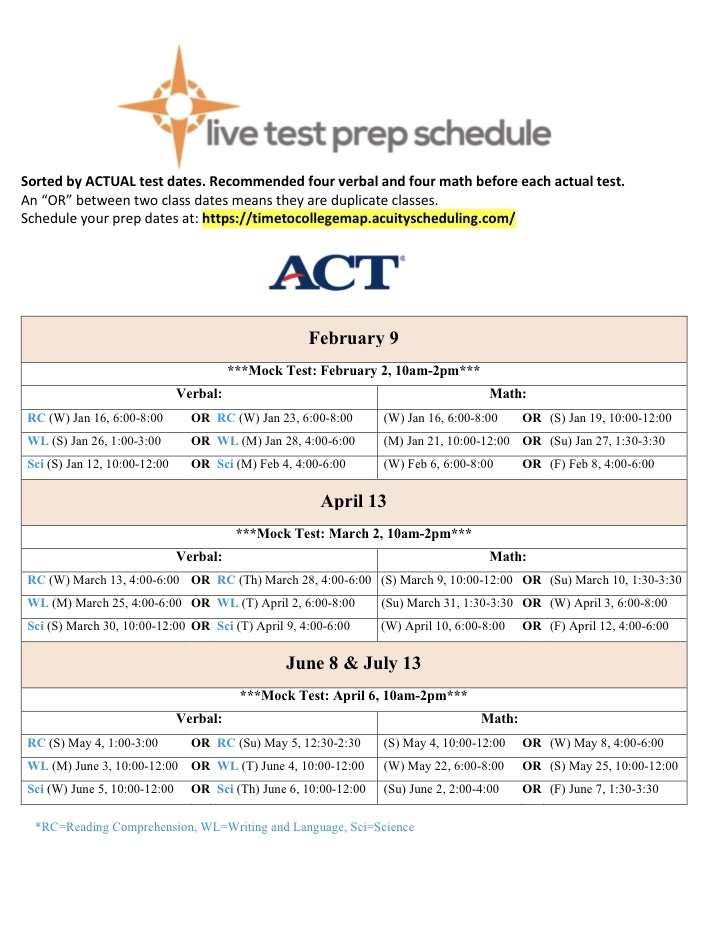table div table+table+table+table+table+table+table+table+table+table+table+table+table+table+table+table+table+table+table+table+table+table+table+table+table+table+table+table+table+table+table+table+table+table+table+table+table div table{width:100%;padding:0}table div table+table+table+table+table+table+table+table+table+table+table+table+table+table+table+table+table+table+table+table+table+table+table+table+table+table+table+table+table+table+table+table+table+table+table+table+table div table img{width:96.23%;padding:0;float:none}table div table+table+table+table+table+table+table+table+table+table+table+table+table+table+table+table+table+table+table+table+table+table+table+table+table+table+table+table+table+table+table+table+table+table+table+table+table div table td{width:100%;padding:0 1.88% 18px}/* styles */## JLV Scholarships!Great Resource for January 2019 Scholarships
Attending college can be quite expensive for students and their families. Luckily, there are many college scholarships and contests available to help pay for a college education. Students should seek out and apply for scholarships in which they meet the eligibility requirements. Click here for January 2019 due dates.

 table div table+table+table+table+table+table+table+table+table+table+table+table+table+table+table+table+table+table+table+table+table+table+table+table+table+table+table+table+table+table+table+table+table+table+table+table+table+table+table+table div table{width:100%;padding:0}table div table+table+table+table+table+table+table+table+table+table+table+table+table+table+table+table+table+table+table+table+table+table+table+table+table+table+table+table+table+table+table+table+table+table+table+table+table+table+table+table div table img{width:96.23%;padding:0;float:none}table div table+table+table+table+table+table+table+table+table+table+table+table+table+table+table+table+table+table+table+table+table+table+table+table+table+table+table+table+table+table+table+table+table+table+table+table+table+table+table+table div table td{width:100%;padding:0 1.88% 18px}/* styles */## Now What?Now What?

Seniors, as you wrap up your applications, you'll certainly feel like celebrating! But, this is no time to let down your guard. Colleges may communicate with you as they work through the admissions process. Pay close attention to:

Temporary student portal

It's a good idea to check these tools daily or at least several times a week! Click for more Additional Information!

 table div table+table+table+table+table+table+table+table+table+table+table+table+table+table+table+table+table+table+table+table+table+table+table+table+table+table+table+table+table+table+table+table+table+table+table+table+table+table+table+table+table+table+table div table{width:100%;padding:0}table div table+table+table+table+table+table+table+table+table+table+table+table+table+table+table+table+table+table+table+table+table+table+table+table+table+table+table+table+table+table+table+table+table+table+table+table+table+table+table+table+table+table+table div table img{width:96.23%;padding:0;float:none}table div table+table+table+table+table+table+table+table+table+table+table+table+table+table+table+table+table+table+table+table+table+table+table+table+table+table+table+table+table+table+table+table+table+table+table+table+table+table+table+table+table+table+table div table td{width:100%;padding:0 1.88% 18px}/* styles */## DEFFERALS and WAITLISTS!DEFERRALS and WAITLISTS:

Being deferred can mean a wide variety of things. In most cases, the college has not completed its review of your file and is “deferring” their decision to a later date. Deferrals typically fall into two categories:

You applied under the Early Action or Early Decision plan and have been pushed back into the regular pool. This may be frustrating but also has an advantage. If you are accepted into the college/university under regular decision, you are not obligated to attend as you would have been if you were accepted under an Early Decision plan (Early Action is non-binding to begin with). You may feel free to consider offers from other schools.
You have applied under a regular decision or rolling admission and the college/university would like to have more information in order to make a decision about your application. In almost every case, a college or university would like to see more grades from the senior year or new test scores. If a school receives the information they want, they could admit you earlier.

Being waitlisted is unlike being deferred; the college has finished reviewing your file and made a decision to put you on a waiting list for admission.

Being on a waitlist typically means that you are placed within a “holding pattern” of sorts. The admissions committee may or may not admit students from the waitlist. And unlike a deferral situation, new information does not usually change a waitlist decision.
What you can do!

 table div table+table+table+table+table+table+table+table+table+table+table+table+table+table+table+table+table+table+table+table+table+table+table+table+table+table+table+table+table+table+table+table+table+table+table+table+table+table+table+table+table+table+table+table+table+table div table{width:100%;padding:0}table div table+table+table+table+table+table+table+table+table+table+table+table+table+table+table+table+table+table+table+table+table+table+table+table+table+table+table+table+table+table+table+table+table+table+table+table+table+table+table+table+table+table+table+table+table+table div table img{width:96.23%;padding:0;float:none}table div table+table+table+table+table+table+table+table+table+table+table+table+table+table+table+table+table+table+table+table+table+table+table+table+table+table+table+table+table+table+table+table+table+table+table+table+table+table+table+table+table+table+table+table+table+table div table td{width:100%;padding:0 1.88% 18px}/* styles */## Scholarships Search Tools

 /* styles */ There are many good scholarship search tools/engines on the internet. Their primary function is to assist you in finding scholarships that match the eligibility criteria you enter for GPA, gender, residency, ethnicity/heritage, religion and area of study, hobbies, interests — some of the most common criteria used. The College Map team recommends the following search engines: Scholarships.com FastWeb Big Future Unigo SallieMae Please make sure to "click" or fill out as much information as possible. Once you've entered your information into the database, the search engine will generate a list of all current scholarships you qualify for. We recommend sorting that list by due date.
 table div table+table+table+table+table+table+table+table+table+table+table+table+table+table+table+table+table+table+table+table+table+table+table+table+table+table+table+table+table+table+table+table+table+table+table+table+table+table+table+table+table+table+table+table+table+table+table+table+table div table{width:100%;padding:0}table div table+table+table+table+table+table+table+table+table+table+table+table+table+table+table+table+table+table+table+table+table+table+table+table+table+table+table+table+table+table+table+table+table+table+table+table+table+table+table+table+table+table+table+table+table+table+table+table+table div table img{width:96.23%;padding:0;float:none}table div table+table+table+table+table+table+table+table+table+table+table+table+table+table+table+table+table+table+table+table+table+table+table+table+table+table+table+table+table+table+table+table+table+table+table+table+table+table+table+table+table+table+table+table+table+table+table+table+table div table td{width:100%;padding:0 1.88% 18px}/* styles */# SENIORS! Institutional Scholarships

Institutional Scholarships are merit-based financial awards provided to you through the college/university you have applied to. Many of your schools will automatically consider you for scholarships, but please make sure that you are checking your individual school's websites for any additional freshman scholarships you may be eligible for that require a separate application. Several schools like, Auburn, Carnegie Mellon, Stetson and Alabama offer NON-automatic consideration scholarships.
We welcome seniors to attend boot camp on Wednesdays to work on scholarships after you have submitted your applications.

 table div table+table+table+table+table+table+table+table+table+table+table+table+table+table+table+table+table+table+table+table+table+table+table+table+table+table+table+table+table+table+table+table+table+table+table+table+table+table+table+table+table+table+table+table+table+table+table+table+table+table+table div table{width:100%;padding:0}table div table+table+table+table+table+table+table+table+table+table+table+table+table+table+table+table+table+table+table+table+table+table+table+table+table+table+table+table+table+table+table+table+table+table+table+table+table+table+table+table+table+table+table+table+table+table+table+table+table+table+table div table img{width:96.23%;padding:0;float:none}table div table+table+table+table+table+table+table+table+table+table+table+table+table+table+table+table+table+table+table+table+table+table+table+table+table+table+table+table+table+table+table+table+table+table+table+table+table+table+table+table+table+table+table+table+table+table+table+table+table+table+table div table td{width:100%;padding:0 1.88% 18px}/* styles */Our private "Your Path" page contains details for upcoming deadlines, events, and important announcements. We encourage you to check it regularly! The password is case-sensitive:
prepare@TCM2018

# Test Prep Schedules

Be sure to register for your spring test prep classes, available on the Your Path page and the Acuity Scheduler. Registration is mandatory; walk-ins may not be able to be accommodated.

# Suggestions?

Is there something you'd like to see in our newsletter? An event you'd like us to host? A friend you'd like to refer? Let us know by simply replying to this message.

 /* styles */ Kind regards, Your Team at The College Map